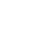Live chatIn mathematics and statistics in general, this principle is applicable in almost every aspect. This is because it has become the new tool for making the best decisions based on identifying and evaluating evidence in a systematic manner. In fact, it has been widely described as a reflective way of thinking that enables to decide on what actions to undertake or which facts to believe. The fundamental idea, however, is that critical thinking is very comprehensive in conceptualizing and analyzing ideas with a view to making a sound decision. However, critical thinking applies certain concepts like confidence interval, margin of error among others in determining how accurate the assumptions made could be (Sumner, 1906).

## Buy Free Custom «Mathematics and Statistics» Essay Paper paper online

* Final order price might be slightly different depending on the current exchange rate of chosen payment system.

Confidence interval is a theoretical degree of confidence levels that the decisions made based on critical thinking fall within acceptable limits, with regards to related cases. For instance, measuring of confidence interval would mean that data related to the phenomenon is collected and analyzed for consistency. However, one has to repeat similar measurements before they arrive at a value that is widely acceptable as a confidence interval. It must be noted, however, that confidence interval does not provide an accurate prediction of the actual value of the parameters under measurements. All it does is to give the approximate probability that the data obtained will be reproducible (Sumner, 1906).

Stay ConnectedMargin of error, on the other hand, is the measure of error that occurred in sample collection for the purpose of survey. As such, if the margin of error is too big, there is less confidence that the value under investigation falls close to the expected value. This technique is mostly used for huge samples where it is impossible to consider the entire population in the statistical determination. Generally, a margin of error would only occur if the population under investigation is not completely sampled. As such, a small portion that has been investigated is used as the reference point for the level of accuracy or conformity to the normal value (Sumner, 1906).

In statistics, these measurements are expressed in terms of percentages for ease of mathematical manipulations. For instance, when a margin of error is adopted for a particular survey, it applies for all values or data that result from the particular survey. Thus, it acts as a reference point from which the authenticity or accuracy of related values are determined. However, large margins of error are likely to mislead because it is ideally hard to determine the accuracy and suitability of the value as the preferred value (Sumner, 1906).

This literature can be used to examine the following problems:

Use Formula 7-2 on P. 336 to determine if the sample size is proportionate or not. Here is that formula: n= [zα/2]2 phat * qhat/ E2 . You'll plug in the phat and qhat values and margin of error you found earlier in the assignment.

For example, in this case, n= [32α/2]2 3 * 8/ 1.962

Limited time Offer

0
0
days
:
0
0
hours
:
0
0
minutes
:
0
0
seconds
Get 19% OFF

Thus, n= 1599.33

To respond to this we could also use sample size calculations to inform our response. Use formula 7-3 on P. 336:

n= [zα/2]2 .25/ E2    = 1665.97

In conclusion, critical thinking is a very important tool in decision making. It ideally enables one to determine how accurate his or her assumptions could be with regards to the data under investigation.

0

Preparing Orders

0

Active Writers

0

Support Agents

Limited offerget 15% off your 1st order